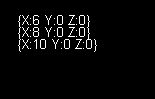# FlatRedBall.Math.PositionedObjectList.SortXInsertionAscending

## Introduction

The SortXInsertionAscending (and SortYInsertionAscending/SortZInsertionAscending) function can be used to sort all contained PositionedObjects in a list by their X (or Y/Z) value.

## Code Example

The following code creates 3 Circles and places them in a PositionedObjectList in descending X order. Then SortXInsertionAscending is called, and the positions of the Circles are printed out:

```FlatRedBall.Math.PositionedObjectList<Circle> circleList =
new FlatRedBall.Math.PositionedObjectList<Circle>();

// Let's create 3 Circles.  Their X values will
// be in decreasing order
Circle first = new Circle();
first.X = 10;

Circle second = new Circle();
second.X = 8;

Circle third = new Circle();
third.X = 6;